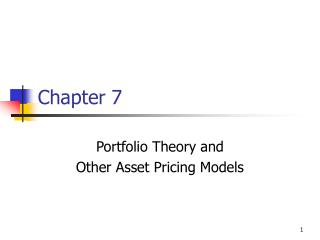Download PresentationChapter 7

Loading in 2 Seconds...

# Chapter 7 - PowerPoint PPT Presentation

Chapter 7. Portfolio Theory and Other Asset Pricing Models. Topics in Chapter. Portfolio Theory Capital Asset Pricing Model (CAPM) Capital Market Line (CML) Security Market Line (SML). Efficient Set. Expected Portfolio Return, r p. Feasible Set. Risk,  p.I am the owner, or an agent authorized to act on behalf of the owner, of the copyrighted work described.
Download Presentation## Chapter 7

An Image/Link below is provided (as is) to download presentation

Download Policy: Content on the Website is provided to you AS IS for your information and personal use and may not be sold / licensed / shared on other websites without getting consent from its author.While downloading, if for some reason you are not able to download a presentation, the publisher may have deleted the file from their server.

- - - - - - - - - - - - - - - - - - - - - - - - - - E N D - - - - - - - - - - - - - - - - - - - - - - - - - -
Presentation Transcript
1. Chapter 7 Portfolio Theory and Other Asset Pricing Models

2. Topics in Chapter • Portfolio Theory • Capital Asset Pricing Model (CAPM) • Capital Market Line (CML) • Security Market Line (SML)

3. Efficient Set Expected Portfolio Return, rp Feasible Set Risk, p Feasible and Efficient Portfolios

4. Feasible and Efficient Portfolios • The feasible set of portfolios represents all portfolios that can be constructed from a given set of stocks. • An efficient portfolio is one that offers: • the most return for a given amount of risk, or • the least risk for a give amount of return. • The collection of efficient portfolios is called the efficient set or efficient frontier.

5. Optimal Portfolios IB2 IB1 Expected Return, rp Optimal Portfolio Investor B IA2 IA1 Optimal Portfolio Investor A Risk p

6. Indifference Curves • Indifference curves reflect an investor’s attitude toward risk as reflected in his or her risk/return tradeoff function. They differ among investors because of differences in risk aversion. • An investor’s optimal portfolio is defined by the tangency point between the efficient set and the investor’s indifference curve.

7. What is the CAPM? • The CAPM is an equilibrium model that specifies the relationship between risk and required rate of return for assets held in well-diversified portfolios. • It is based on the premise that only one factor affects risk. • What is that factor?

8. What impact does rRF have onthe efficient frontier? • When a risk-free asset is added to the feasible set, investors can create portfolios that combine this asset with a portfolio of risky assets. • The straight line connecting rRF with M, the tangency point between the line and the old efficient set, becomes the new efficient frontier.

9. Expected Return, rp Z . B M ^ . rM . The Capital Market Line (CML): New Efficient Set A rRF M Risk, p Efficient Set with a Risk-Free Asset

10. What is the Capital Market Line? • The Capital Market Line (CML) is all linear combinations of the risk-free asset and Portfolio M. • Portfolios below the CML are inferior. • The CML defines the new efficient set. • All investors will choose a portfolio on the CML.

11. ^ rM - rRF ^ p. rp = rRF + M Intercept Slope Risk measure The CML Equation

12. What does the CML tell us? • The expected rate of return on any efficient portfolio is equal to the risk-free rate plus a risk premium. • The optimal portfolio for any investor is the point of tangency between the CML and the investor’s indifference curves.

13. I2 Expected Return, rp CML I1 . M . ^ rM R ^ rR R = Optimal Portfolio rRF Risk, p R M Capital Market Line

14. What is the Security Market Line (SML)? • The CML gives the risk/return relationship for efficient portfolios. • The Security Market Line (SML), also part of the CAPM, gives the risk/return relationship for individual stocks.

15. The SML Equation • The measure of risk used in the SML is the beta coefficient of company i, bi. • The SML equation: ri = rRF + (RPM) bi

16. How are betas calculated? • Run a regression line of past returns on Stock i versus returns on the market. • The regression line is called the characteristic line. • The slope coefficient of the characteristic line is defined as the beta coefficient.

17. CAPM Required Return for Stock i CAPM: ri = rRF + (rM - rRF)bi ri = 6.8% + (6.3%)(0.9) = 12.47%